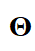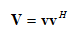# {complex affine} .* {convex}

The relationship between V and ThetaisTheta is the variable to be optimized by the algorithm, and V is the auxiliary variable.

Here is my code.
cvx_solver mosek
cvx_begin

``````variable Theta(2,2)
expressions V(3,3) v(3,1)
v=[diag(Theta).' 1].';

f1=log(2).*(trace(H_ak1*V)*ap1+wk(1,1)*ap1+noise);
f2=log(2).*(trace(H_ak2*V)*ap2+wk(1,2)*ap2+noise);
f3=log(2).*(trace(H_ak3*V)*ap3+wk(1,2)*ap3+noise);
ge1=log(2).*(trace(H_ae*V)*(ap1-p_k0(1,1))+we*(ap1-p_k0(1,1))+noise);
ge2=log(2).*(trace(H_ae*V)*(ap2-p_k0(1,2))+we*(ap2-p_k0(1,2))+noise);
ge3=log(2).*(trace(H_ae*V)*(ap3-p_k0(1,3))+we*(ap3-p_k0(1,3))+noise);

der_g1=((ap1-p_k0(1,1))*H_ak1'*log2(exp(1)))/(trace(H_ak1*V0)*(ap1-p_k0(1,1))+wk(1,1)*(ap1-p_k0(1,1))+noise);
der_g2=((ap2-p_k0(1,2))*H_ak2'*log2(exp(1)))/(trace(H_ak2*V0)*(ap2-p_k0(1,2))+wk(1,2)*(ap2-p_k0(1,2))+noise);
der_g3=((ap3-p_k0(1,3))*H_ak3'*log2(exp(1)))/(trace(H_ak3*V0)*(ap3-p_k0(1,3))+wk(1,3)*(ap3-p_k0(1,3))+noise);
g1_ub=log(2).*(trace(H_ak1*V0)*(ap1-p_k0(1,1))+wk(1,1)*(ap1-p_k0(1,1))+noise)+trace(der_g1'*(V-V0));
g2_ub=log(2).*(trace(H_ak2*V0)*(ap2-p_k0(1,2))+wk(1,2)*(ap2-p_k0(1,2))+noise)+trace(der_g2'*(V-V0));
g3_ub=log(2).*(trace(H_ak3*V0)*(ap3-p_k0(1,3))+wk(1,3)*(ap3-p_k0(1,3))+noise)+trace(der_g3'*(V-V0));
``````

The running result is

Disciplined convex programming error:
Cannot perform the operation: {complex affine} .* {convex}

z = feval( oper, x, y );

f1=log(2).(trace(H_ak1V)*ap1+wk(1,1)*ap1+noise);

If my code is written as follows, it will run successfully, but I can’t get the theta I actually want to optimize Theta from the optimized V.

cvx_solver mosek
cvx_begin
variable V(3,3)

``````f1=log(2).*(trace(H_ak1*V)*ap1+wk(1,1)*ap1+noise);
f2=log(2).*(trace(H_ak2*V)*ap2+wk(1,2)*ap2+noise);
f3=log(2).*(trace(H_ak3*V)*ap3+wk(1,2)*ap3+noise);``````

`expressions V(3,3) v(3,1)` indicated V is V(3,3), but `V=quad_form(v,eye(3))` indicated V is V(1). Check your code carefully. And your code is not complete. It is hard to help. pls check http://web.cvxr.com/cvx/doc/funcref.html#arithmetic-operators

Please provide reproducible code, complete with input data. I don’t see why that error message should occur.

it doesn’t cause any harm, but the expression declarations aren’t needed, because the expressions are created in a single command, rather than by individual element. In fact, the next 2 lines override those expression declarations, and create `v` as 3 by 1 (mixed affine and constant) and `V` as scalar convex expression. So `f1` looks to me like `V` is the only cvx expression on the RHS, and therefore I don’t see how the error can occur; unless you are not showing us some portion of the code, and one or more of the other things on the RHS is a CVX expression (complex affine) rather than only input data.

I’m sorry I didn’t provide the complete code.
There is my complete code.
b_k_H=g_exp_rk’*diag(G);
h_ak_H=[b_k_H h_exp_ak.’];

b_e_H=g_exp_re’*diag(G);
h_ae_H=[b_e_H h_exp_ae];

H_ak1=h_ak_H(1,:)’*h_ak_H(1,:);
H_ak2=h_ak_H(2,:)’*h_ak_H(2,:);
H_ak3=h_ak_H(3,:)’*h_ak_H(3,:);
H_ae=h_ae_H’*h_ae_H;

theta0=diag(Theta0);
v0=[theta0.’ 1].’;
V0=v0*v0’;

ap1=p_k0(1,1)+a_ij0(2,1)*p_k0(1,2)+a_ij0(3,1)*p_k0(1,3);
ap2=a_ij0(1,2)*p_k0(1,1)+p_k0(1,2)+a_ij0(3,2)*p_k0(1,3);
ap3=a_ij0(1,3)*p_k0(1,1)+a_ij0(2,3)*p_k0(1,2)+p_k0(1,3);

wk=zeros(1,K);
for n=1:K
wk(1,n)=(PL-Z)/power(sqrt((norm(q0-Pk(n,:)))^2+H1^2),FL)+t_k(1,n)/power(sqrt((norm(q0-u))^2+(H1-H2)^2),2);
end
we=(PL-Z)/power(sqrt((norm(q0-Pe))^2+H1^2),FL)+t_e/power(sqrt((norm(q0-u))^2+(H1-H2)^2),2);

cvx_solver mosek
cvx_begin

``````variable Theta(2,2)
expressions V(3,3) v(3,1)
v=[diag(Theta).' 1].';

f1=log(2).*(trace(H_ak1*V)*ap1+wk(1,1)*ap1+noise);
f2=log(2).*(trace(H_ak2*V)*ap2+wk(1,2)*ap2+noise);
f3=log(2).*(trace(H_ak3*V)*ap3+wk(1,2)*ap3+noise);
ge1=log(2).*(trace(H_ae*V)*(ap1-p_k0(1,1))+we*(ap1-p_k0(1,1))+noise);
ge2=log(2).*(trace(H_ae*V)*(ap2-p_k0(1,2))+we*(ap2-p_k0(1,2))+noise);
ge3=log(2).*(trace(H_ae*V)*(ap3-p_k0(1,3))+we*(ap3-p_k0(1,3))+noise);

der_g1=((ap1-p_k0(1,1))*H_ak1'*log2(exp(1)))/(trace(H_ak1*V0)*(ap1-p_k0(1,1))+wk(1,1)*(ap1-p_k0(1,1))+noise);
der_g2=((ap2-p_k0(1,2))*H_ak2'*log2(exp(1)))/(trace(H_ak2*V0)*(ap2-p_k0(1,2))+wk(1,2)*(ap2-p_k0(1,2))+noise);
der_g3=((ap3-p_k0(1,3))*H_ak3'*log2(exp(1)))/(trace(H_ak3*V0)*(ap3-p_k0(1,3))+wk(1,3)*(ap3-p_k0(1,3))+noise);
g1_ub=log(2).*(trace(H_ak1*V0)*(ap1-p_k0(1,1))+wk(1,1)*(ap1-p_k0(1,1))+noise)+trace(der_g1'*(V-V0));
g2_ub=log(2).*(trace(H_ak2*V0)*(ap2-p_k0(1,2))+wk(1,2)*(ap2-p_k0(1,2))+noise)+trace(der_g2'*(V-V0));
g3_ub=log(2).*(trace(H_ak3*V0)*(ap3-p_k0(1,3))+wk(1,3)*(ap3-p_k0(1,3))+noise)+trace(der_g3'*(V-V0));

der_fe1=ap1*H_ae'*log2(exp(1))/(trace(H_ae*V0)*ap1+we*ap1+noise);
der_fe2=ap2*H_ae'*log2(exp(1))/(trace(H_ae*V0)*ap2+we*ap2+noise);
der_fe3=ap3*H_ae'*log2(exp(1))/(trace(H_ae*V0)*ap3+we*ap3+noise);
fe1_ub=log(2).*(trace(H_ae*V0)*ap1+we*ap1+noise)+trace(der_fe1'*(V-V0));
fe2_ub=log(2).*(trace(H_ae*V0)*ap2+we*ap2+noise)+trace(der_fe2'*(V-V0));
fe3_ub=log(2).*(trace(H_ae*V0)*ap3+we*ap3+noise)+trace(der_fe3'*(V-V0));

[evec,eval]=eig(V0);
evalues=diag(eval);
[a,b]=sort(evalues,'descend');
evectors=evec(:,b);
V0_max_vector=evectors(:,1);

V0_exp=norm(V0,2)+trace(V0_max_vector*V0_max_vector'*(V-V0));
Punish_V=norm_nuc(V)-real(V0_exp);
result2=-(real((g1_ub-f1-ge1+fe1_ub)+(g2_ub-f2-ge2+fe2_ub)+(g3_ub-f3-ge3+fe3_ub))+m_V*Punish_V);

maximize result2

subject to
diag(V)==ones(3,1);%(35b)
lambda_min(V)>=0;
``````

cvx_end
H_ak and H_ae are 3x3 complex matrixs.

Thanks. I find my code wrong. I don’t know how to writein CVX.
If I write V=v*v’; in CVX.
Matlab said Only scalar quadratic forms can be specified in CVX.

Again, you should read the CVX guide carefully about every function you use. And your problem to me is like you’re doing SDP relaxation, though you didn’t show it completely to us.

You don’t. Perhaps whatever paper you got this from expects you to use a semidefinite relaxation
` [V v;v' 1] >= 0` (if in sdp mode)

1 Like